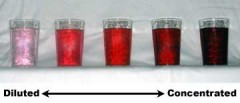# How Is Density Related To Concentration?Concentration is the amount of substance present in a given quantity of another substance. It is thus a quantity or number of atoms present in a unit volume of another substance. The first substance could be solid, liquid or gas. This is called a solute and the substance in which it is added is called solvent. This solvent is generally liquid.

Density is defined as mass per unit volume of a substance. Generally, the density of an object is the total mass of the object divided by the total volume of the object. This object is generally a solid or liquid. Thus, density and concentration are related terms dealing with concentration in general. Concentration deals with small particle while density is concerned with the mass. Thus, concentration is directly proportional to density for elemental substances but not in general.
thanked the writer.
Higher concentration means more mass of solute in the solution. And since solutes overall add negligible increase in volume to the solution, density increases.

So, higher concentration => higher density;
thanked the writer.
The density of a substance is the mass per unit of volume, or the mass divided by the volume. It's sort of like how tightly packed the particles are in one substance. Concentration involves solutions--how much solute is dissolved in a given amount of solvent. You can make a concentrated solution of Kool Aid by adding lots of Kool Aid Mix and lots of sugar. You can make a dilute solution of Kool Aid by adding only a little bit of Kool Aid Mix and water.
thanked the writer.

I think they are directly proportional to each other.

thanked the writer.